EK不够快？再学个Dinic吧

2018-07-28 13:41:43

# 特别感谢：@ComeIntoPower提出了许多修改建议

## 什么是Dinic？

Dinic算法（又称Dinitz算法）是一个在网络流中计算最大流的强多项式复杂度的算法，设想由以色列（前苏联）的计算机科学家Yefim (Chaim) A. Dinitz在1970年提出。

# Dinic讲解

Dinic算法分为两个步骤：

1. bfs分层(在EK中bfs是用于寻找增广路的)
2. dfs增广(dfs？EK中貌似没有这玩意啊，确定能高效？)
3. 咦！刚才不是说两个步骤吗？重复执行1.2.直到图中无增广路为止

bool bfs(){
memset(dep,0x3f,sizeof(dep));
memset(inque,0,sizeof(inque));
dep[s]=0;
queue<int>q;
q.push(s);
while(!q.empty()){
int u=q.front();
q.pop();
inque[u]=0;
int d=node[i].v;
if(dep[d]>dep[u]+1&&node[i].val){
dep[d]=dep[u]+1;//注意与EK的区别
if(inque[d]==0){
q.push(d);
inque[d]=1;
}
}
}
}
if(dep[t]!=0x3f3f3f3f)return 1;
return 0;
}//给增广路上的点分层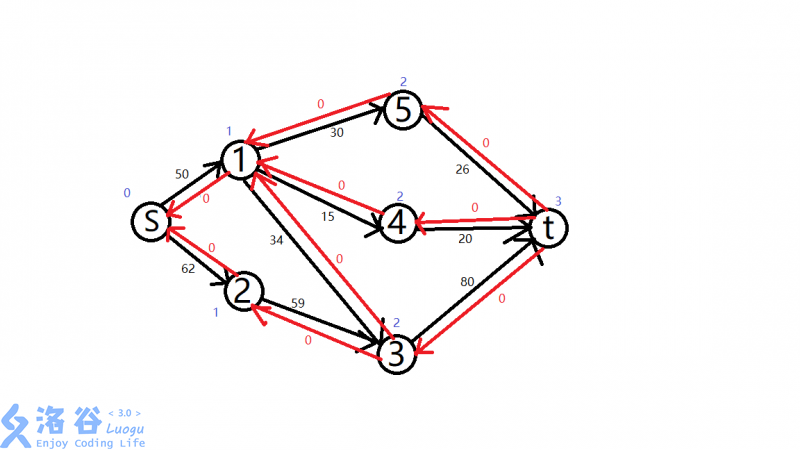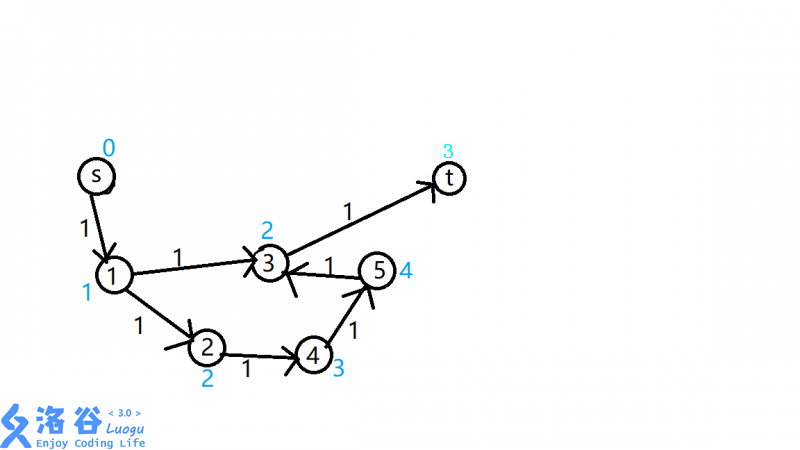int dfs(int u,int flow){//u是当前点，low是当前增广路上的最小边权
int rlow=0;
if(u==t)return flow;//已经到达t可以用当前路径
int d=node[i].v;
if(node[i].val&&dep[d]==dep[u]+1){//寻找最短可增广路径
if(rlow=dfs(d,min(flow,node[i].val))){
node[i].val-=rlow;
node[i^1].val+=rlow;
//正向边加流，反向边减流，思想同EK
return rlow;
}
}
}
return 0;//无法到达t，无增广路
}//寻找增广路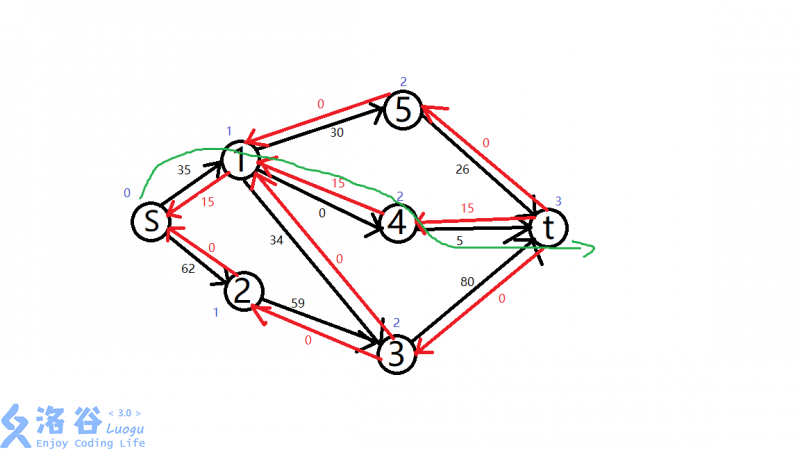s->1->5->t: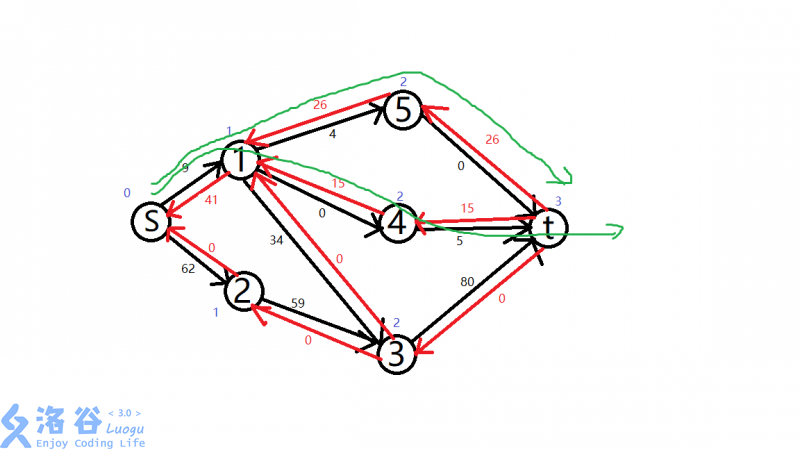s->1->3->t: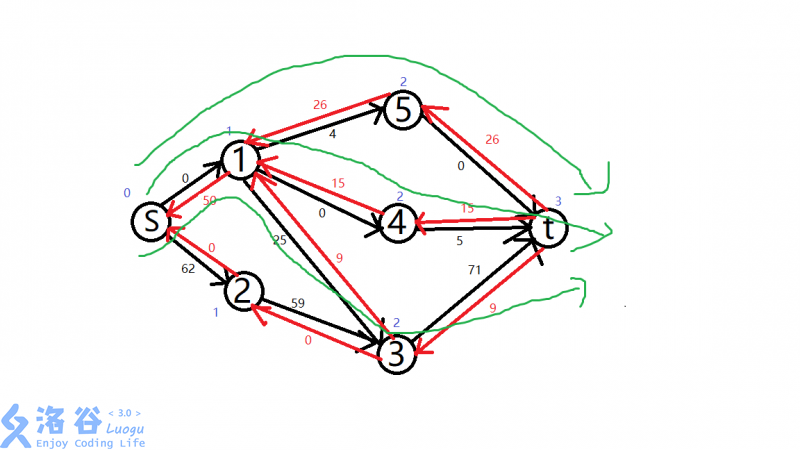s->2->3->t: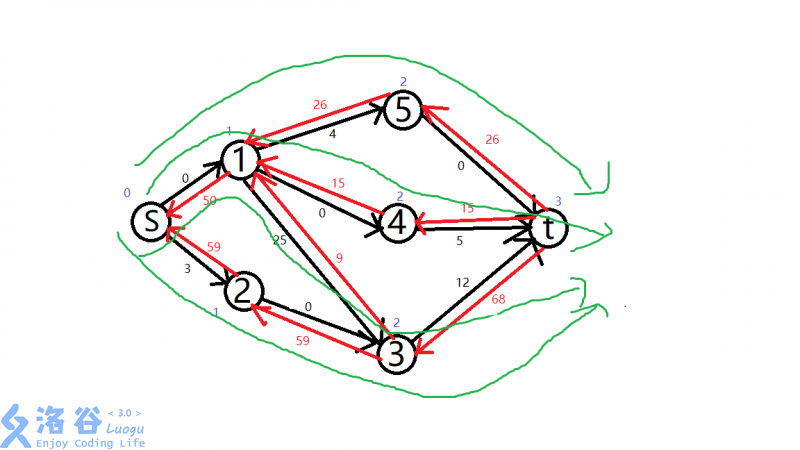//Dinic网络最大流模板
#include<iostream>
#include<cstdio>
#include<cstring>
#include<algorithm>
#include<queue>
using namespace std;
const int inf=1<<30;
int top=1;
int maxflow=0;
int n,m,s,t;
struct Node{
int v;
int val;
int next;
}node;
inline void addedge(int u,int v,int val){
node[++top].v=v;
node[top].val=val;
}
int x=0;char c=getchar();
while(c>'9'||c<'0')c=getchar();
while(c>='0'&&c<='9')x=x*10+c-'0',c=getchar();
return x;
}
bool bfs(){
memset(dep,0x3f,sizeof(dep));
memset(inque,0,sizeof(inque));
dep[s]=0;
queue<int>q;
q.push(s);
while(!q.empty()){
int u=q.front();
q.pop();
inque[u]=0;
int d=node[i].v;
if(dep[d]>dep[u]+1&&node[i].val){
dep[d]=dep[u]+1;//注意与EK的区别
if(inque[d]==0){
q.push(d);
inque[d]=1;
}
}
}
}
if(dep[t]!=0x3f3f3f3f)return 1;
return 0;
}//给增广路上的点分层
inline int min(int x,int y){
return x<y?x:y;
}
int dfs(int u,int flow){
int rlow=0;
if(u==t)return flow;
int d=node[i].v;
if(node[i].val&&dep[d]==dep[u]+1){
if(rlow=dfs(d,min(flow,node[i].val))){
node[i].val-=rlow;
node[i^1].val+=rlow;
return rlow;
}
}
}
return 0;
}//寻找增广路
int Dinic(){
int lowflow;
while(bfs()){
while(lowflow=dfs(s,inf))maxflow+=lowflow;
}
return maxflow;
}//Dinic寻找最大流
int main(){
register int i;
int u,v,val;
printf("%d",Dinic());
return 0;
}

## 优化：

int dfs(int u,int flow){
int rlow=0;
if(u==t){
vis==1;//可以到达t
maxflow+=flow;//其实可以直接在这里累加最大流
return flow;
}
int used=0;//该点已经使用的流量
int d=node[i].v;
if(node[i].val&&dep[d]==dep[u]+1){
if(rlow=dfs(d,min(flow-used,node[i].val))){
used+=rlow;//该点使用的流量增加
node[i].val-=rlow;
node[i^1].val+=rlow;
if(used==flow)break;//该点流量满了，没必要再找了
}
}
}
return used;//返回该点已使用流量
}//寻找增广路 

int Dinic(){
while(bfs()){
vis=1;
while(vis==1){
vis=0;
dfs(s,inf);
}
}
return maxflow;
}//Dinic寻找最大流 


int Dinic(){
while(bfs()){
dfs(s,inf);
}
return maxflow;
}//Dinic寻找最大流 

## 优化2.0：（传说中的当前弧优化）

memset(dep,0x3f,sizeof(dep));
memset(inque,0,sizeof(inque));

for(register int i=0;i<=n;i++)cur[i]=head[i],dep[i]=0x3f3f3f3f,inque[i]=0;
//两个memset一个memcpy还不如直接循环

## 在dfs中

for(int i=head[u];i;i=node[i].next){
int d=node[i].v;
if(node[i].val&&dep[d]==dep[u]+1){
if(rlow=dfs(d,min(flow-used,node[i].val))){
used+=rlow;
node[i].val-=rlow;
node[i^1].val+=rlow;
if(used==flow)break;
}
}
}

for(int i=cur[u];i;i=node[i].next){
cur[u]=i;//修改当前弧
int d=node[i].v;
if(node[i].val&&dep[d]==dep[u]+1){
if(rlow=dfs(d,min(flow-uesd,node[i].val))){
used+=rlow;
node[i].val-=rlow;
node[i^1].val+=rlow;
if(used==flow)break;
}
}
}

# 感谢观看

dist[d]==dist[u]+node[i].w

int dfs(int u,int flow){
if(u==t){vis[t]=1;maxflow+=flow;return flow;}//可以到达t，加流
int used=0;//该点使用了的流量
vis[u]=1;
int d=node[i].v;
if((vis[d]==0||d==t)&&node[i].val!=0&&dist[d]==dist[u]+node[i].w){
int minflow=dfs(d,min(flow-used,node[i].val));
if(minflow!=0)cost+=node[i].w*minflow,node[i].val-=minflow,node[i^1].val+=minflow,used+=minflow;
//可以到达t，加费用，扣流量
if(used==flow)break;//小小的优化
}
}
return used;
}

int mincostmaxflow(){//最小费用最大流
while(spfa()){
vis[t]=1;
while(vis[t]){//能到达t就继续
memset(vis,0,sizeof(vis));//由于是数组，要memset
dfs(s,inf);
}
}
return maxflow;
}

#include<iostream>
#include<cstring>
#include<algorithm>
#include<queue>
#include<cstdio>
using namespace std;
const int inf=1<<30;
int n,m,s,t;
int cost,maxflow;
int vis;//是否到达过该点
int dist;//到t的单位费用
struct Node{
int val;
int v;
int next;
int w;
}node;
int x=0;
char c=getchar();
while(c>'9'||c<'0')c=getchar();
while(c>='0'&&c<='9')x=x*10+c-'0',c=getchar();
return x;
}
inline void addedge(int u,int v,int val,int w){
node[++top].v=v;
node[top].w=w;
node[top].val=val;
}
bool spfa(){
memset(vis,0,sizeof(vis));
memset(dist,0x3f,sizeof(dist));
dist[s]=0;
vis[s]=1;
queue<int>q;
q.push(s);
while(!q.empty()){
int u=q.front();
q.pop();
vis[u]=0;
int d=node[i].v;
if(dist[d]>dist[u]+node[i].w&&node[i].val){
dist[d]=dist[u]+node[i].w;
if(vis[d]==0){
q.push(d);
vis[d]=1;
}
}
}
}
return dist[t]!=0x3f3f3f3f;
}//spfa同EK
inline int min(int x,int y){
return x<y?x:y;
}
int dfs(int u,int flow){
if(u==t){vis[t]=1;maxflow+=low;return flow;}//可以到达t，加流
int used=0;//该条路径可用流量
vis[u]=1;
int d=node[i].v;
if((vis[d]==0||d==t)&&node[i].val!=0&&dist[d]==dist[u]+node[i].w){
int minflow=dfs(d,min(flow-used,node[i].val));
if(minflow!=0)cost+=node[i].w*minflow,node[i].val-=minflow,node[i^1].val+=minflow,used+=minflow;
//可以到达t，加费用，扣流量
if(used==flow)break;
}
}
return used;
}
int mincostmaxflow(){
while(spfa()){
vis[t]=1;
while(vis[t]){
memset(vis,0,sizeof(vis));
dfs(s,inf);
}
}
return maxflow;
}
int main(){
int u,v,w,val;
register int i;
for(i=1;i<=m;i++){
}
mincostmaxflow();
printf("%d %d",maxflow,cost);
return 0;
}

EK（还吸了氧气的）

## 欢迎各位dalao提出修改建议

ISAP和HLPP已经写好了（作者快累死了）

• star
首页